Next: Calculating the smoothness of Up: Smoothness estimation Previous: Smoothness a la SPM

### A more robust smoothness estimator

The estimator described in section 2.2.1 becomes increasingly inaccurate for sub voxel width filter sizes. As the filter width decreases equation 12 becomes increasingly inaccurate until the estimation in equation  14 is no longer valid. An alternative estimate  offers a more robust result when evaluating images with small spatial correlation. Consider the following definitions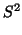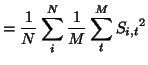(16)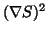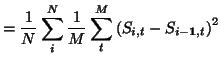(17)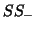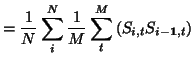(18)

where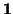is a unit vector along the dimension currently under consideration.is the individual voxel variance,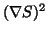the variance of the difference between each voxel and its edgewise neighbours, andis the correlation of two neighbouring voxels.

The approach outlined in 2.2.1 calculates the quantity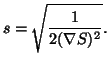(19)

Forman et al derive the alternate formula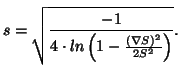(20)

Mark Jenkinson  has provided an extensive derivation for this formula as well as pointing out another, computationally cleaner, variation on the same theme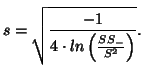(21)

Equations 20 and 21 remain accurate for relatively small spatial smoothnesses and it is equation 21 that you will find in the FSL code base.Next: Calculating the smoothness of Up: Smoothness estimation Previous: Smoothness a la SPM
David Flitney 2001-11-29